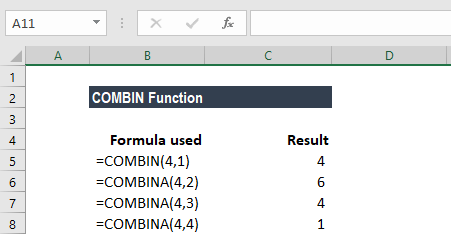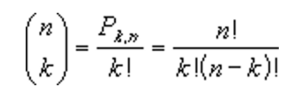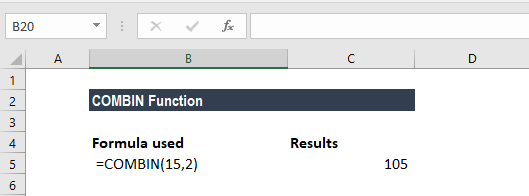# COMBIN Function

Calculates the number of combinations without repetitions for a given number of items

## What is the COMBIN Function?

The COMBIN Function is an Excel Math and Trigonometry function. The function will calculate the number of combinations without repetitions for a given number of items. It was introduced in MS Excel 2000.

### Formula

=COMBIN(number, number_chosen)

The COMBIN function uses the following arguments:

1. Number (required argument) – The number should either be greater than or equal to 0. Also, it should be greater than or equal to the number_chosen. Non-integer values, if entered for this parameter, are truncated.
2. Number_chosen (required argument) – This is the number of items in each of the combinations. It must be greater than or equal to 0. Non-integer values are truncated.

### How to use the COMBIN Function in Excel?

To understand the uses of this function, let’s consider a few examples:

#### Example 1

Suppose we are given six numbers: 1, 2, 3, 4, 5, 6. Now let’s see how the COMBIN function calculates the number of combinations (without repetitions) for the other numbers of objects taken from a set of 4.For any six objects such as “a, b, c, d, e, f,” there are 15 different combinations (without repetitions) of two objects. These are:

ab, ac, ad, ae, af, bc, bd, be, bf, cd, ce, cf, de, df, ef

The COMBIN Function helps to derive such combinations as shown above. The number of combinations is as follows, where number = n and number_chosen = k:#### Example 2

Let’s now see how we can use this function in day-to-day life. Suppose there are 15 students and we wish to pair them into two-person teams. Using the COMBIN function, we can see the possible two-person teams that can be formed from 15 students.As seen above, 105 combinations without repetitions are possible.

### Difference between the COMBIN and COMBINA Functions

The COMBIN and COMBINA functions differ in the following ways:

• COMBIN does not count repetitions, whereas COMBINA does. For example, in a set of three objects – a, b, c – COMBIN would return the result 3; the combinations are ab, ac, bc. Whereas, COMBINA would return the result 6; the combinations are aa, ab, ac, bb, bc, cc.
• The formulas used by both functions are different.

### Things to remember about the COMBIN Function

1. #VALUE! error – Occurs when either of the arguments is non-numeric.
2. #NUM! error – Occurs when the value of either argument is outside of its constraint.
3. When we speak about a combination, it is any set or subset of items, regardless of its internal order. Combinations are separate from permutations, as the internal order is significant for permutations.

Thanks for reading CFI’s guide to important Excel functions! By taking the time to learn and master these functions, you’ll significantly speed up your financial analysis. To learn more, check out these additional CFI resources:

• Excel Functions for Finance